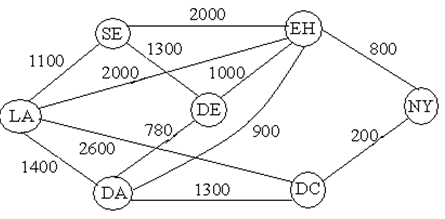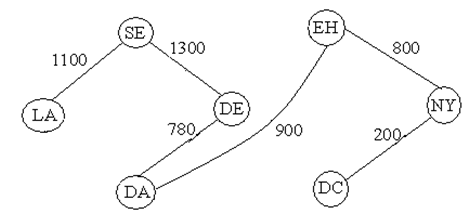#### Minimal Spanning Tree Problem, Prim’s Algorithm

Minimal Spanning Tree Problem

A tree can be defined as an acyclic, undirected and connected graph. A spanning tree is a subgraph of G, that is, undirected and connected graph, is a tree and consists of all the vertices of G. A minimum spanning tree is a spanning tree but has weights or lengths related with edges and the total weight is at the minimum.

Prim's Algorithm

• It begins at any vertex (say A) in a graph and recognizes the least cost vertex (say B) linked to the start vertex.
• Now either from A or B, it will recognize the next least costly vertex relation, without creating cycle (say C)
• Now either from A, B or C recognize the other least costly vertex relation, without creating a cycle and so on.
• Ultimately all the vertices will be linked without any cycles and a minimum spanning treewill be the outcome.

Sample Assignment

Assume that it is desired to set up a cable communication network that connects major cities, which is revealed in the figure. Find out how the cities are linked such that the total cable mileage is reduced.C = {LA}                                                        C' = {SE, DE, DA, EH, NY, DC}

C = {LA, SE}                                                 C' = {DE, DA, EH, NY, DC}

C = {LA, SE, DE}                                         C' = {DA, EH, NY, DC}

C = {LA, SE, DE, DA}                                 C' = {EH, NY, DC}

C = {LA, SE, DE, DA, EH}                          C' = {NY, DC}

C = {LA, SE, DE, DA, EH, NY}                  C' = {DC}

C = {LA, SE, DE, DA, EH, NY, DC}          C' = { }

The resulting network isTherefore the total cable mileage is 1100 + 1300 + 780 + 900 + 800 + 200 = 5080